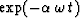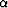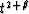Next: Noise, surface waves and Up: COSMETIC ASPECT OF WAVE Previous: COSMETIC ASPECT OF WAVE

## t squared

Echos get weaker with time. To be able to see the data at late times, we generally increase data amplification with time. I have rarely been disappointed by my choice of the function t2 for the scaling factor. The t2 scaling function cannot always be expected to work, because it is based on a very simple model. But I find t2 to be more satisfactory than a popular alternative, the growing exponential. The function t2 has no parameters whereas the exponential function requires two parameters, one for the time constant, and one for the time at which you must stop the exponential because it gets too large.

The first of the two powers of t arises because we are transforming three dimensions to one. The seismic waves are spreading out in three dimensions, and the surface area on the expanding spherical wave increases in proportion to the radius squared. Thus the area on which the energy is distributed is increasing in proportion to time squared. But seismic amplitudes are proportional to the square root of the energy. So the basic geometry of energy spreading predicts only a single power of time for the spherical divergence correction.

An additional power of t arises from a simple absorption calculation. Absorption requires a model. The model I'll propose is too simple to explain everything about seismic absorption, but it nicely predicts the extra power of t that experience shows we need. For the model we assume:

 1 One dimensional propagation 2 Constant velocity 3 Constant absorption Q-1 4 Reflection coefficients random in depth 5 No multiple reflections 6 White source

These assumptions immediately tell us that a monochromatic wave would decrease exponentially with depth, say, aswhere t is travel-time depth andis a decay constant which is inversely proportional to the wave quality factor Q. Many people go astray when they model real seismic data by such a monochromatic wave. A better model is that the seismic source is broad band, for example an impulse function. Because of absorption, high frequencies decay rapidly, eventually leaving only low frequencies, hence a lower signal strength. At propagation time t the original white (constant) spectrum is replaced by the forementioned functionwhich is a damped exponential function of frequency. The seismic energy available for the creation of an impulsive time function is just proportional to the area under the damped exponential function of frequency. As for the phase, all frequencies will be in phase because the source is assumed impulsive and the velocity is assumed constant. Integrating the exponential from zero to infinite frequency provides us with an inverse power of t thus completing the justification of a t2 divergence correction.

It is curious that the shape of the expected seismogram envelope t-2 does not depend on the dissipation constant.But changing the spectrum of the seismic source does change the shape of the envelope. It is left for an exercise to show that a seismic source with spectrumimplies a divergence correction.

The seismic velocity increases with depth, so sometimes people who know the velocity may improve the divergence correction by making it a function of velocity (and hence offset) as well as time.

In reality it may be fortuitous that t2 fits data so well. Actually, Q generally increases with depth whereas reflection coefficients generally decrease with depth.Next: Noise, surface waves and Up: COSMETIC ASPECT OF WAVE Previous: COSMETIC ASPECT OF WAVE
Stanford Exploration Project
10/31/1997